# Solve 2

Solve integer equation: a +b+c =30

a, b, c = can be odd natural number from this set (1,3,5,7,9,11,13,15)

Result

P =  0

#### Solution:The equation has the following integer solutions:
a+b+c=30
##### a1=-10, b1=50, c1=-10a2=-10, b2=49, c2=-9a3=-10, b3=48, c3=-8a4=-10, b4=47, c4=-7a5=-10, b5=46, c5=-6a6=-10, b6=45, c6=-5a7=-10, b7=44, c7=-4a8=-10, b8=43, c8=-3a9=-10, b9=42, c9=-2a10=-10, b10=41, c10=-1a11=-10, b11=40, c11=0a12=-10, b12=39, c12=1a13=-10, b13=38, c13=2a14=-10, b14=37, c14=3a15=-10, b15=36, c15=4a16=-10, b16=35, c16=5a17=-10, b17=34, c17=6a18=-10, b18=33, c18=7a19=-10, b19=32, c19=8a20=-10, b20=31, c20=9a21=-10, b21=30, c21=10a22=-10, b22=29, c22=11a23=-10, b23=28, c23=12a24=-10, b24=27, c24=13a25=-10, b25=26, c25=14a26=-10, b26=25, c26=15a27=-10, b27=24, c27=16a28=-10, b28=23, c28=17a29=-10, b29=22, c29=18a30=-10, b30=21, c30=19a31=-10, b31=20, c31=20a32=-10, b32=19, c32=21a33=-10, b33=18, c33=22a34=-10, b34=17, c34=23a35=-10, b35=16, c35=24a36=-10, b36=15, c36=25a37=-10, b37=14, c37=26a38=-10, b38=13, c38=27a39=-10, b39=12, c39=28a40=-10, b40=11, c40=29a41=-10, b41=10, c41=30a42=-10, b42=9, c42=31a43=-10, b43=8, c43=32a44=-10, b44=7, c44=33a45=-10, b45=6, c45=34a46=-10, b46=5, c46=35a47=-10, b47=4, c47=36a48=-10, b48=3, c48=37a49=-10, b49=2, c49=38a50=-10, b50=1, c50=39a51=-10, b51=0, c51=40a52=-10, b52=-1, c52=41a53=-10, b53=-2, c53=42a54=-10, b54=-3, c54=43a55=-10, b55=-4, c55=44a56=-10, b56=-5, c56=45a57=-10, b57=-6, c57=46a58=-10, b58=-7, c58=47a59=-10, b59=-8, c59=48a60=-10, b60=-9, c60=49a61=-10, b61=-10, c61=50a62=-9, b62=49, c62=-10a63=-9, b63=48, c63=-9a64=-9, b64=47, c64=-8a65=-9, b65=46, c65=-7a66=-9, b66=45, c66=-6a67=-9, b67=44, c67=-5a68=-9, b68=43, c68=-4a69=-9, b69=42, c69=-3a70=-9, b70=41, c70=-2a71=-9, b71=40, c71=-1a72=-9, b72=39, c72=0a73=-9, b73=38, c73=1a74=-9, b74=37, c74=2a75=-9, b75=36, c75=3a76=-9, b76=35, c76=4a77=-9, b77=34, c77=5a78=-9, b78=33, c78=6a79=-9, b79=32, c79=7a80=-9, b80=31, c80=8a81=-9, b81=30, c81=9a82=-9, b82=29, c82=10a83=-9, b83=28, c83=11a84=-9, b84=27, c84=12a85=-9, b85=26, c85=13a86=-9, b86=25, c86=14a87=-9, b87=24, c87=15a88=-9, b88=23, c88=16a89=-9, b89=22, c89=17a90=-9, b90=21, c90=18a91=-9, b91=20, c91=19a92=-9, b92=19, c92=20a93=-9, b93=18, c93=21a94=-9, b94=17, c94=22a95=-9, b95=16, c95=23a96=-9, b96=15, c96=24a97=-9, b97=14, c97=25a98=-9, b98=13, c98=26a99=-9, b99=12, c99=27a100=-9, b100=11, c100=28a101=-9, b101=10, c101=29a102=-9, b102=9, c102=30a103=-9, b103=8, c103=31a104=-9, b104=7, c104=32a105=-9, b105=6, c105=33a106=-9, b106=5, c106=34a107=-9, b107=4, c107=35a108=-9, b108=3, c108=36a109=-9, b109=2, c109=37a110=-9, b110=1, c110=38a111=-9, b111=0, c111=39a112=-9, b112=-1, c112=40a113=-9, b113=-2, c113=41a114=-9, b114=-3, c114=42a115=-9, b115=-4, c115=43a116=-9, b116=-5, c116=44a117=-9, b117=-6, c117=45a118=-9, b118=-7, c118=46a119=-9, b119=-8, c119=47a120=-9, b120=-9, c120=48a121=-9, b121=-10, c121=49a122=-8, b122=48, c122=-10a123=-8, b123=47, c123=-9a124=-8, b124=46, c124=-8a125=-8, b125=45, c125=-7a126=-8, b126=44, c126=-6a127=-8, b127=43, c127=-5a128=-8, b128=42, c128=-4a129=-8, b129=41, c129=-3a130=-8, b130=40, c130=-2a131=-8, b131=39, c131=-1a132=-8, b132=38, c132=0a133=-8, b133=37, c133=1a134=-8, b134=36, c134=2a135=-8, b135=35, c135=3a136=-8, b136=34, c136=4a137=-8, b137=33, c137=5a138=-8, b138=32, c138=6a139=-8, b139=31, c139=7a140=-8, b140=30, c140=8a141=-8, b141=29, c141=9a142=-8, b142=28, c142=10a143=-8, b143=27, c143=11a144=-8, b144=26, c144=12a145=-8, b145=25, c145=13a146=-8, b146=24, c146=14a147=-8, b147=23, c147=15a148=-8, b148=22, c148=16a149=-8, b149=21, c149=17a150=-8, b150=20, c150=18a151=-8, b151=19, c151=19a152=-8, b152=18, c152=20a153=-8, b153=17, c153=21a154=-8, b154=16, c154=22a155=-8, b155=15, c155=23a156=-8, b156=14, c156=24a157=-8, b157=13, c157=25a158=-8, b158=12, c158=26a159=-8, b159=11, c159=27a160=-8, b160=10, c160=28a161=-8, b161=9, c161=29a162=-8, b162=8, c162=30a163=-8, b163=7, c163=31a164=-8, b164=6, c164=32a165=-8, b165=5, c165=33a166=-8, b166=4, c166=34a167=-8, b167=3, c167=35a168=-8, b168=2, c168=36a169=-8, b169=1, c169=37a170=-8, b170=0, c170=38a171=-8, b171=-1, c171=39a172=-8, b172=-2, c172=40a173=-8, b173=-3, c173=41a174=-8, b174=-4, c174=42a175=-8, b175=-5, c175=43a176=-8, b176=-6, c176=44a177=-8, b177=-7, c177=45a178=-8, b178=-8, c178=46a179=-8, b179=-9, c179=47a180=-8, b180=-10, c180=48a181=-7, b181=47, c181=-10a182=-7, b182=46, c182=-9a183=-7, b183=45, c183=-8a184=-7, b184=44, c184=-7a185=-7, b185=43, c185=-6a186=-7, b186=42, c186=-5a187=-7, b187=41, c187=-4a188=-7, b188=40, c188=-3a189=-7, b189=39, c189=-2a190=-7, b190=38, c190=-1a191=-7, b191=37, c191=0a192=-7, b192=36, c192=1a193=-7, b193=35, c193=2a194=-7, b194=34, c194=3a195=-7, b195=33, c195=4a196=-7, b196=32, c196=5a197=-7, b197=31, c197=6a198=-7, b198=30, c198=7a199=-7, b199=29, c199=8a200=-7, b200=28, c200=9a201=-7, b201=27, c201=10a202=-7, b202=26, c202=11a203=-7, b203=25, c203=12a204=-7, b204=24, c204=13a205=-7, b205=23, c205=14a206=-7, b206=22, c206=15a207=-7, b207=21, c207=16a208=-7, b208=20, c208=17a209=-7, b209=19, c209=18a210=-7, b210=18, c210=19a211=-7, b211=17, c211=20a212=-7, b212=16, c212=21a213=-7, b213=15, c213=22a214=-7, b214=14, c214=23a215=-7, b215=13, c215=24a216=-7, b216=12, c216=25a217=-7, b217=11, c217=26a218=-7, b218=10, c218=27a219=-7, b219=9, c219=28a220=-7, b220=8, c220=29a221=-7, b221=7, c221=30a222=-7, b222=6, c222=31a223=-7, b223=5, c223=32a224=-7, b224=4, c224=33a225=-7, b225=3, c225=34a226=-7, b226=2, c226=35a227=-7, b227=1, c227=36a228=-7, b228=0, c228=37a229=-7, b229=-1, c229=38a230=-7, b230=-2, c230=39a231=-7, b231=-3, c231=40a232=-7, b232=-4, c232=41a233=-7, b233=-5, c233=42a234=-7, b234=-6, c234=43a235=-7, b235=-7, c235=44a236=-7, b236=-8, c236=45a237=-7, b237=-9, c237=46a238=-7, b238=-10, c238=47a239=-6, b239=46, c239=-10a240=-6, b240=45, c240=-9a241=-6, b241=44, c241=-8a242=-6, b242=43, c242=-7a243=-6, b243=42, c243=-6a244=-6, b244=41, c244=-5a245=-6, b245=40, c245=-4a246=-6, b246=39, c246=-3a247=-6, b247=38, c247=-2a248=-6, b248=37, c248=-1a249=-6, b249=36, c249=0a250=-6, b250=35, c250=1a251=-6, b251=34, c251=2a252=-6, b252=33, c252=3a253=-6, b253=32, c253=4a254=-6, b254=31, c254=5a255=-6, b255=30, c255=6a256=-6, b256=29, c256=7a257=-6, b257=28, c257=8a258=-6, b258=27, c258=9a259=-6, b259=26, c259=10a260=-6, b260=25, c260=11a261=-6, b261=24, c261=12a262=-6, b262=23, c262=13a263=-6, b263=22, c263=14a264=-6, b264=21, c264=15a265=-6, b265=20, c265=16a266=-6, b266=19, c266=17a267=-6, b267=18, c267=18a268=-6, b268=17, c268=19a269=-6, b269=16, c269=20a270=-6, b270=15, c270=21a271=-6, b271=14, c271=22a272=-6, b272=13, c272=23a273=-6, b273=12, c273=24a274=-6, b274=11, c274=25a275=-6, b275=10, c275=26a276=-6, b276=9, c276=27a277=-6, b277=8, c277=28a278=-6, b278=7, c278=29a279=-6, b279=6, c279=30a280=-6, b280=5, c280=31a281=-6, b281=4, c281=32a282=-6, b282=3, c282=33a283=-6, b283=2, c283=34a284=-6, b284=1, c284=35a285=-6, b285=0, c285=36a286=-6, b286=-1, c286=37a287=-6, b287=-2, c287=38a288=-6, b288=-3, c288=39a289=-6, b289=-4, c289=40a290=-6, b290=-5, c290=41a291=-6, b291=-6, c291=42a292=-6, b292=-7, c292=43a293=-6, b293=-8, c293=44a294=-6, b294=-9, c294=45a295=-6, b295=-10, c295=46a296=-5, b296=45, c296=-10a297=-5, b297=44, c297=-9a298=-5, b298=43, c298=-8a299=-5, b299=42, c299=-7a300=-5, b300=41, c300=-6a301=-5, b301=40, c301=-5a302=-5, b302=39, c302=-4a303=-5, b303=38, c303=-3a304=-5, b304=37, c304=-2a305=-5, b305=36, c305=-1a306=-5, b306=35, c306=0a307=-5, b307=34, c307=1a308=-5, b308=33, c308=2a309=-5, b309=32, c309=3a310=-5, b310=31, c310=4a311=-5, b311=30, c311=5a312=-5, b312=29, c312=6a313=-5, b313=28, c313=7a314=-5, b314=27, c314=8a315=-5, b315=26, c315=9a316=-5, b316=25, c316=10a317=-5, b317=24, c317=11a318=-5, b318=23, c318=12a319=-5, b319=22, c319=13a320=-5, b320=21, c320=14a321=-5, b321=20, c321=15a322=-5, b322=19, c322=16a323=-5, b323=18, c323=17a324=-5, b324=17, c324=18a325=-5, b325=16, c325=19a326=-5, b326=15, c326=20a327=-5, b327=14, c327=21a328=-5, b328=13, c328=22a329=-5, b329=12, c329=23a330=-5, b330=11, c330=24a331=-5, b331=10, c331=25a332=-5, b332=9, c332=26a333=-5, b333=8, c333=27a334=-5, b334=7, c334=28a335=-5, b335=6, c335=29a336=-5, b336=5, c336=30a337=-5, b337=4, c337=31a338=-5, b338=3, c338=32a339=-5, b339=2, c339=33a340=-5, b340=1, c340=34a341=-5, b341=0, c341=35a342=-5, b342=-1, c342=36a343=-5, b343=-2, c343=37a344=-5, b344=-3, c344=38a345=-5, b345=-4, c345=39a346=-5, b346=-5, c346=40a347=-5, b347=-6, c347=41a348=-5, b348=-7, c348=42a349=-5, b349=-8, c349=43a350=-5, b350=-9, c350=44a351=-5, b351=-10, c351=45a352=-4, b352=44, c352=-10a353=-4, b353=43, c353=-9a354=-4, b354=42, c354=-8a355=-4, b355=41, c355=-7a356=-4, b356=40, c356=-6a357=-4, b357=39, c357=-5a358=-4, b358=38, c358=-4a359=-4, b359=37, c359=-3a360=-4, b360=36, c360=-2a361=-4, b361=35, c361=-1a362=-4, b362=34, c362=0a363=-4, b363=33, c363=1a364=-4, b364=32, c364=2a365=-4, b365=31, c365=3a366=-4, b366=30, c366=4a367=-4, b367=29, c367=5a368=-4, b368=28, c368=6a369=-4, b369=27, c369=7a370=-4, b370=26, c370=8a371=-4, b371=25, c371=9a372=-4, b372=24, c372=10a373=-4, b373=23, c373=11a374=-4, b374=22, c374=12a375=-4, b375=21, c375=13a376=-4, b376=20, c376=14a377=-4, b377=19, c377=15a378=-4, b378=18, c378=16a379=-4, b379=17, c379=17a380=-4, b380=16, c380=18a381=-4, b381=15, c381=19a382=-4, b382=14, c382=20a383=-4, b383=13, c383=21a384=-4, b384=12, c384=22a385=-4, b385=11, c385=23a386=-4, b386=10, c386=24a387=-4, b387=9, c387=25a388=-4, b388=8, c388=26a389=-4, b389=7, c389=27a390=-4, b390=6, c390=28a391=-4, b391=5, c391=29a392=-4, b392=4, c392=30a393=-4, b393=3, c393=31a394=-4, b394=2, c394=32a395=-4, b395=1, c395=33a396=-4, b396=0, c396=34a397=-4, b397=-1, c397=35a398=-4, b398=-2, c398=36a399=-4, b399=-3, c399=37a400=-4, b400=-4, c400=38a401=-4, b401=-5, c401=39a402=-4, b402=-6, c402=40a403=-4, b403=-7, c403=41a404=-4, b404=-8, c404=42a405=-4, b405=-9, c405=43a406=-4, b406=-10, c406=44a407=-3, b407=43, c407=-10a408=-3, b408=42, c408=-9a409=-3, b409=41, c409=-8a410=-3, b410=40, c410=-7a411=-3, b411=39, c411=-6a412=-3, b412=38, c412=-5a413=-3, b413=37, c413=-4a414=-3, b414=36, c414=-3a415=-3, b415=35, c415=-2a416=-3, b416=34, c416=-1a417=-3, b417=33, c417=0a418=-3, b418=32, c418=1a419=-3, b419=31, c419=2a420=-3, b420=30, c420=3a421=-3, b421=29, c421=4a422=-3, b422=28, c422=5a423=-3, b423=27, c423=6a424=-3, b424=26, c424=7a425=-3, b425=25, c425=8a426=-3, b426=24, c426=9a427=-3, b427=23, c427=10a428=-3, b428=22, c428=11a429=-3, b429=21, c429=12a430=-3, b430=20, c430=13a431=-3, b431=19, c431=14a432=-3, b432=18, c432=15a433=-3, b433=17, c433=16a434=-3, b434=16, c434=17a435=-3, b435=15, c435=18a436=-3, b436=14, c436=19a437=-3, b437=13, c437=20a438=-3, b438=12, c438=21a439=-3, b439=11, c439=22a440=-3, b440=10, c440=23a441=-3, b441=9, c441=24a442=-3, b442=8, c442=25a443=-3, b443=7, c443=26a444=-3, b444=6, c444=27a445=-3, b445=5, c445=28a446=-3, b446=4, c446=29a447=-3, b447=3, c447=30a448=-3, b448=2, c448=31a449=-3, b449=1, c449=32a450=-3, b450=0, c450=33a451=-3, b451=-1, c451=34a452=-3, b452=-2, c452=35a453=-3, b453=-3, c453=36a454=-3, b454=-4, c454=37a455=-3, b455=-5, c455=38a456=-3, b456=-6, c456=39a457=-3, b457=-7, c457=40a458=-3, b458=-8, c458=41a459=-3, b459=-9, c459=42a460=-3, b460=-10, c460=43a461=-2, b461=42, c461=-10a462=-2, b462=41, c462=-9a463=-2, b463=40, c463=-8a464=-2, b464=39, c464=-7a465=-2, b465=38, c465=-6a466=-2, b466=37, c466=-5a467=-2, b467=36, c467=-4a468=-2, b468=35, c468=-3a469=-2, b469=34, c469=-2a470=-2, b470=33, c470=-1a471=-2, b471=32, c471=0a472=-2, b472=31, c472=1a473=-2, b473=30, c473=2a474=-2, b474=29, c474=3a475=-2, b475=28, c475=4a476=-2, b476=27, c476=5a477=-2, b477=26, c477=6a478=-2, b478=25, c478=7a479=-2, b479=24, c479=8a480=-2, b480=23, c480=9a481=-2, b481=22, c481=10a482=-2, b482=21, c482=11a483=-2, b483=20, c483=12a484=-2, b484=19, c484=13a485=-2, b485=18, c485=14a486=-2, b486=17, c486=15a487=-2, b487=16, c487=16a488=-2, b488=15, c488=17a489=-2, b489=14, c489=18a490=-2, b490=13, c490=19a491=-2, b491=12, c491=20a492=-2, b492=11, c492=21a493=-2, b493=10, c493=22a494=-2, b494=9, c494=23a495=-2, b495=8, c495=24a496=-2, b496=7, c496=25a497=-2, b497=6, c497=26a498=-2, b498=5, c498=27a499=-2, b499=4, c499=28a500=-2, b500=3, c500=29a501=-2, b501=2, c501=30a502=-2, b502=1, c502=31a503=-2, b503=0, c503=32a504=-2, b504=-1, c504=33a505=-2, b505=-2, c505=34a506=-2, b506=-3, c506=35a507=-2, b507=-4, c507=36a508=-2, b508=-5, c508=37a509=-2, b509=-6, c509=38a510=-2, b510=-7, c510=39a511=-2, b511=-8, c511=40a512=-2, b512=-9, c512=41a513=-2, b513=-10, c513=42a514=-1, b514=41, c514=-10a515=-1, b515=40, c515=-9a516=-1, b516=39, c516=-8a517=-1, b517=38, c517=-7a518=-1, b518=37, c518=-6a519=-1, b519=36, c519=-5a520=-1, b520=35, c520=-4a521=-1, b521=34, c521=-3a522=-1, b522=33, c522=-2a523=-1, b523=32, c523=-1a524=-1, b524=31, c524=0a525=-1, b525=30, c525=1a526=-1, b526=29, c526=2a527=-1, b527=28, c527=3a528=-1, b528=27, c528=4a529=-1, b529=26, c529=5a530=-1, b530=25, c530=6a531=-1, b531=24, c531=7a532=-1, b532=23, c532=8a533=-1, b533=22, c533=9a534=-1, b534=21, c534=10a535=-1, b535=20, c535=11a536=-1, b536=19, c536=12a537=-1, b537=18, c537=13a538=-1, b538=17, c538=14a539=-1, b539=16, c539=15a540=-1, b540=15, c540=16a541=-1, b541=14, c541=17a542=-1, b542=13, c542=18a543=-1, b543=12, c543=19a544=-1, b544=11, c544=20a545=-1, b545=10, c545=21a546=-1, b546=9, c546=22a547=-1, b547=8, c547=23a548=-1, b548=7, c548=24a549=-1, b549=6, c549=25a550=-1, b550=5, c550=26a551=-1, b551=4, c551=27a552=-1, b552=3, c552=28a553=-1, b553=2, c553=29a554=-1, b554=1, c554=30a555=-1, b555=0, c555=31a556=-1, b556=-1, c556=32a557=-1, b557=-2, c557=33a558=-1, b558=-3, c558=34a559=-1, b559=-4, c559=35a560=-1, b560=-5, c560=36a561=-1, b561=-6, c561=37a562=-1, b562=-7, c562=38a563=-1, b563=-8, c563=39a564=-1, b564=-9, c564=40a565=-1, b565=-10, c565=41a566=0, b566=40, c566=-10a567=0, b567=39, c567=-9a568=0, b568=38, c568=-8a569=0, b569=37, c569=-7a570=0, b570=36, c570=-6a571=0, b571=35, c571=-5a572=0, b572=34, c572=-4a573=0, b573=33, c573=-3a574=0, b574=32, c574=-2a575=0, b575=31, c575=-1a576=0, b576=30, c576=0a577=0, b577=29, c577=1a578=0, b578=28, c578=2a579=0, b579=27, c579=3a580=0, b580=26, c580=4a581=0, b581=25, c581=5a582=0, b582=24, c582=6a583=0, b583=23, c583=7a584=0, b584=22, c584=8a585=0, b585=21, c585=9a586=0, b586=20, c586=10a587=0, b587=19, c587=11a588=0, b588=18, c588=12a589=0, b589=17, c589=13a590=0, b590=16, c590=14a591=0, b591=15, c591=15a592=0, b592=14, c592=16a593=0, b593=13, c593=17a594=0, b594=12, c594=18a595=0, b595=11, c595=19a596=0, b596=10, c596=20a597=0, b597=9, c597=21a598=0, b598=8, c598=22a599=0, b599=7, c599=23a600=0, b600=6, c600=24a601=0, b601=5, c601=25a602=0, b602=4, c602=26a603=0, b603=3, c603=27a604=0, b604=2, c604=28a605=0, b605=1, c605=29a606=0, b606=0, c606=30a607=0, b607=-1, c607=31a608=0, b608=-2, c608=32a609=0, b609=-3, c609=33a610=0, b610=-4, c610=34a611=0, b611=-5, c611=35a612=0, b612=-6, c612=36a613=0, b613=-7, c613=37a614=0, b614=-8, c614=38a615=0, b615=-9, c615=39a616=0, b616=-10, c616=40a617=1, b617=39, c617=-10a618=1, b618=38, c618=-9a619=1, b619=37, c619=-8a620=1, b620=36, c620=-7a621=1, b621=35, c621=-6a622=1, b622=34, c622=-5a623=1, b623=33, c623=-4a624=1, b624=32, c624=-3a625=1, b625=31, c625=-2a626=1, b626=30, c626=-1a627=1, b627=29, c627=0a628=1, b628=28, c628=1a629=1, b629=27, c629=2a630=1, b630=26, c630=3a631=1, b631=25, c631=4a632=1, b632=24, c632=5a633=1, b633=23, c633=6a634=1, b634=22, c634=7a635=1, b635=21, c635=8a636=1, b636=20, c636=9a637=1, b637=19, c637=10a638=1, b638=18, c638=11a639=1, b639=17, c639=12a640=1, b640=16, c640=13a641=1, b641=15, c641=14a642=1, b642=14, c642=15a643=1, b643=13, c643=16a644=1, b644=12, c644=17a645=1, b645=11, c645=18a646=1, b646=10, c646=19a647=1, b647=9, c647=20a648=1, b648=8, c648=21a649=1, b649=7, c649=22a650=1, b650=6, c650=23a651=1, b651=5, c651=24a652=1, b652=4, c652=25a653=1, b653=3, c653=26a654=1, b654=2, c654=27a655=1, b655=1, c655=28a656=1, b656=0, c656=29a657=1, b657=-1, c657=30a658=1, b658=-2, c658=31a659=1, b659=-3, c659=32a660=1, b660=-4, c660=33a661=1, b661=-5, c661=34a662=1, b662=-6, c662=35a663=1, b663=-7, c663=36a664=1, b664=-8, c664=37a665=1, b665=-9, c665=38a666=1, b666=-10, c666=39a667=2, b667=38, c667=-10a668=2, b668=37, c668=-9a669=2, b669=36, c669=-8a670=2, b670=35, c670=-7a671=2, b671=34, c671=-6a672=2, b672=33, c672=-5a673=2, b673=32, c673=-4a674=2, b674=31, c674=-3a675=2, b675=30, c675=-2a676=2, b676=29, c676=-1a677=2, b677=28, c677=0a678=2, b678=27, c678=1a679=2, b679=26, c679=2a680=2, b680=25, c680=3a681=2, b681=24, c681=4a682=2, b682=23, c682=5a683=2, b683=22, c683=6a684=2, b684=21, c684=7a685=2, b685=20, c685=8a686=2, b686=19, c686=9a687=2, b687=18, c687=10a688=2, b688=17, c688=11a689=2, b689=16, c689=12a690=2, b690=15, c690=13a691=2, b691=14, c691=14a692=2, b692=13, c692=15a693=2, b693=12, c693=16a694=2, b694=11, c694=17a695=2, b695=10, c695=18a696=2, b696=9, c696=19a697=2, b697=8, c697=20a698=2, b698=7, c698=21a699=2, b699=6, c699=22a700=2, b700=5, c700=23a701=2, b701=4, c701=24a702=2, b702=3, c702=25a703=2, b703=2, c703=26a704=2, b704=1, c704=27a705=2, b705=0, c705=28a706=2, b706=-1, c706=29a707=2, b707=-2, c707=30a708=2, b708=-3, c708=31a709=2, b709=-4, c709=32a710=2, b710=-5, c710=33a711=2, b711=-6, c711=34a712=2, b712=-7, c712=35a713=2, b713=-8, c713=36a714=2, b714=-9, c714=37a715=2, b715=-10, c715=38a716=3, b716=37, c716=-10a717=3, b717=36, c717=-9a718=3, b718=35, c718=-8a719=3, b719=34, c719=-7a720=3, b720=33, c720=-6a721=3, b721=32, c721=-5a722=3, b722=31, c722=-4a723=3, b723=30, c723=-3a724=3, b724=29, c724=-2a725=3, b725=28, c725=-1a726=3, b726=27, c726=0a727=3, b727=26, c727=1a728=3, b728=25, c728=2a729=3, b729=24, c729=3a730=3, b730=23, c730=4a731=3, b731=22, c731=5a732=3, b732=21, c732=6a733=3, b733=20, c733=7a734=3, b734=19, c734=8a735=3, b735=18, c735=9a736=3, b736=17, c736=10a737=3, b737=16, c737=11a738=3, b738=15, c738=12a739=3, b739=14, c739=13a740=3, b740=13, c740=14a741=3, b741=12, c741=15a742=3, b742=11, c742=16a743=3, b743=10, c743=17a744=3, b744=9, c744=18a745=3, b745=8, c745=19a746=3, b746=7, c746=20a747=3, b747=6, c747=21a748=3, b748=5, c748=22a749=3, b749=4, c749=23a750=3, b750=3, c750=24a751=3, b751=2, c751=25a752=3, b752=1, c752=26a753=3, b753=0, c753=27a754=3, b754=-1, c754=28a755=3, b755=-2, c755=29a756=3, b756=-3, c756=30a757=3, b757=-4, c757=31a758=3, b758=-5, c758=32a759=3, b759=-6, c759=33a760=3, b760=-7, c760=34a761=3, b761=-8, c761=35a762=3, b762=-9, c762=36a763=3, b763=-10, c763=37a764=4, b764=36, c764=-10a765=4, b765=35, c765=-9a766=4, b766=34, c766=-8a767=4, b767=33, c767=-7a768=4, b768=32, c768=-6a769=4, b769=31, c769=-5a770=4, b770=30, c770=-4a771=4, b771=29, c771=-3a772=4, b772=28, c772=-2a773=4, b773=27, c773=-1a774=4, b774=26, c774=0a775=4, b775=25, c775=1a776=4, b776=24, c776=2a777=4, b777=23, c777=3a778=4, b778=22, c778=4a779=4, b779=21, c779=5a780=4, b780=20, c780=6a781=4, b781=19, c781=7a782=4, b782=18, c782=8a783=4, b783=17, c783=9a784=4, b784=16, c784=10a785=4, b785=15, c785=11a786=4, b786=14, c786=12a787=4, b787=13, c787=13a788=4, b788=12, c788=14a789=4, b789=11, c789=15a790=4, b790=10, c790=16a791=4, b791=9, c791=17a792=4, b792=8, c792=18a793=4, b793=7, c793=19a794=4, b794=6, c794=20a795=4, b795=5, c795=21a796=4, b796=4, c796=22a797=4, b797=3, c797=23a798=4, b798=2, c798=24a799=4, b799=1, c799=25a800=4, b800=0, c800=26a801=4, b801=-1, c801=27a802=4, b802=-2, c802=28a803=4, b803=-3, c803=29a804=4, b804=-4, c804=30a805=4, b805=-5, c805=31a806=4, b806=-6, c806=32a807=4, b807=-7, c807=33a808=4, b808=-8, c808=34a809=4, b809=-9, c809=35a810=4, b810=-10, c810=36a811=5, b811=35, c811=-10a812=5, b812=34, c812=-9a813=5, b813=33, c813=-8a814=5, b814=32, c814=-7a815=5, b815=31, c815=-6a816=5, b816=30, c816=-5a817=5, b817=29, c817=-4a818=5, b818=28, c818=-3a819=5, b819=27, c819=-2a820=5, b820=26, c820=-1a821=5, b821=25, c821=0a822=5, b822=24, c822=1a823=5, b823=23, c823=2a824=5, b824=22, c824=3a825=5, b825=21, c825=4a826=5, b826=20, c826=5a827=5, b827=19, c827=6a828=5, b828=18, c828=7a829=5, b829=17, c829=8a830=5, b830=16, c830=9a831=5, b831=15, c831=10a832=5, b832=14, c832=11a833=5, b833=13, c833=12a834=5, b834=12, c834=13a835=5, b835=11, c835=14a836=5, b836=10, c836=15a837=5, b837=9, c837=16a838=5, b838=8, c838=17a839=5, b839=7, c839=18a840=5, b840=6, c840=19a841=5, b841=5, c841=20a842=5, b842=4, c842=21a843=5, b843=3, c843=22a844=5, b844=2, c844=23a845=5, b845=1, c845=24a846=5, b846=0, c846=25a847=5, b847=-1, c847=26a848=5, b848=-2, c848=27a849=5, b849=-3, c849=28a850=5, b850=-4, c850=29a851=5, b851=-5, c851=30a852=5, b852=-6, c852=31a853=5, b853=-7, c853=32a854=5, b854=-8, c854=33a855=5, b855=-9, c855=34a856=5, b856=-10, c856=35a857=6, b857=34, c857=-10a858=6, b858=33, c858=-9a859=6, b859=32, c859=-8a860=6, b860=31, c860=-7a861=6, b861=30, c861=-6a862=6, b862=29, c862=-5a863=6, b863=28, c863=-4a864=6, b864=27, c864=-3a865=6, b865=26, c865=-2a866=6, b866=25, c866=-1a867=6, b867=24, c867=0a868=6, b868=23, c868=1a869=6, b869=22, c869=2a870=6, b870=21, c870=3a871=6, b871=20, c871=4a872=6, b872=19, c872=5a873=6, b873=18, c873=6a874=6, b874=17, c874=7a875=6, b875=16, c875=8a876=6, b876=15, c876=9a877=6, b877=14, c877=10a878=6, b878=13, c878=11a879=6, b879=12, c879=12a880=6, b880=11, c880=13a881=6, b881=10, c881=14a882=6, b882=9, c882=15a883=6, b883=8, c883=16a884=6, b884=7, c884=17a885=6, b885=6, c885=18a886=6, b886=5, c886=19a887=6, b887=4, c887=20a888=6, b888=3, c888=21a889=6, b889=2, c889=22a890=6, b890=1, c890=23a891=6, b891=0, c891=24a892=6, b892=-1, c892=25a893=6, b893=-2, c893=26a894=6, b894=-3, c894=27a895=6, b895=-4, c895=28a896=6, b896=-5, c896=29a897=6, b897=-6, c897=30a898=6, b898=-7, c898=31a899=6, b899=-8, c899=32a900=6, b900=-9, c900=33a901=6, b901=-10, c901=34a902=7, b902=33, c902=-10a903=7, b903=32, c903=-9a904=7, b904=31, c904=-8a905=7, b905=30, c905=-7a906=7, b906=29, c906=-6a907=7, b907=28, c907=-5a908=7, b908=27, c908=-4a909=7, b909=26, c909=-3a910=7, b910=25, c910=-2a911=7, b911=24, c911=-1a912=7, b912=23, c912=0a913=7, b913=22, c913=1a914=7, b914=21, c914=2a915=7, b915=20, c915=3a916=7, b916=19, c916=4a917=7, b917=18, c917=5a918=7, b918=17, c918=6a919=7, b919=16, c919=7a920=7, b920=15, c920=8a921=7, b921=14, c921=9a922=7, b922=13, c922=10a923=7, b923=12, c923=11a924=7, b924=11, c924=12a925=7, b925=10, c925=13a926=7, b926=9, c926=14a927=7, b927=8, c927=15a928=7, b928=7, c928=16a929=7, b929=6, c929=17a930=7, b930=5, c930=18a931=7, b931=4, c931=19a932=7, b932=3, c932=20a933=7, b933=2, c933=21a934=7, b934=1, c934=22a935=7, b935=0, c935=23a936=7, b936=-1, c936=24a937=7, b937=-2, c937=25a938=7, b938=-3, c938=26a939=7, b939=-4, c939=27a940=7, b940=-5, c940=28a941=7, b941=-6, c941=29a942=7, b942=-7, c942=30a943=7, b943=-8, c943=31a944=7, b944=-9, c944=32a945=7, b945=-10, c945=33a946=8, b946=32, c946=-10a947=8, b947=31, c947=-9a948=8, b948=30, c948=-8a949=8, b949=29, c949=-7a950=8, b950=28, c950=-6a951=8, b951=27, c951=-5a952=8, b952=26, c952=-4a953=8, b953=25, c953=-3a954=8, b954=24, c954=-2a955=8, b955=23, c955=-1a956=8, b956=22, c956=0a957=8, b957=21, c957=1a958=8, b958=20, c958=2a959=8, b959=19, c959=3a960=8, b960=18, c960=4a961=8, b961=17, c961=5a962=8, b962=16, c962=6a963=8, b963=15, c963=7a964=8, b964=14, c964=8a965=8, b965=13, c965=9a966=8, b966=12, c966=10a967=8, b967=11, c967=11a968=8, b968=10, c968=12a969=8, b969=9, c969=13a970=8, b970=8, c970=14a971=8, b971=7, c971=15a972=8, b972=6, c972=16a973=8, b973=5, c973=17a974=8, b974=4, c974=18a975=8, b975=3, c975=19a976=8, b976=2, c976=20a977=8, b977=1, c977=21a978=8, b978=0, c978=22a979=8, b979=-1, c979=23a980=8, b980=-2, c980=24a981=8, b981=-3, c981=25a982=8, b982=-4, c982=26a983=8, b983=-5, c983=27a984=8, b984=-6, c984=28a985=8, b985=-7, c985=29a986=8, b986=-8, c986=30a987=8, b987=-9, c987=31a988=8, b988=-10, c988=32a989=9, b989=31, c989=-10a990=9, b990=30, c990=-9a991=9, b991=29, c991=-8a992=9, b992=28, c992=-7a993=9, b993=27, c993=-6a994=9, b994=26, c994=-5a995=9, b995=25, c995=-4a996=9, b996=24, c996=-3a997=9, b997=23, c997=-2a998=9, b998=22, c998=-1a999=9, b999=21, c999=0a1000=9, b1000=20, c1000=1a1001=9, b1001=19, c1001=2a1002=9, b1002=18, c1002=3a1003=9, b1003=17, c1003=4a1004=9, b1004=16, c1004=5a1005=9, b1005=15, c1005=6a1006=9, b1006=14, c1006=7a1007=9, b1007=13, c1007=8a1008=9, b1008=12, c1008=9a1009=9, b1009=11, c1009=10a1010=9, b1010=10, c1010=11a1011=9, b1011=9, c1011=12a1012=9, b1012=8, c1012=13a1013=9, b1013=7, c1013=14a1014=9, b1014=6, c1014=15a1015=9, b1015=5, c1015=16a1016=9, b1016=4, c1016=17a1017=9, b1017=3, c1017=18a1018=9, b1018=2, c1018=19a1019=9, b1019=1, c1019=20a1020=9, b1020=0, c1020=21a1021=9, b1021=-1, c1021=22a1022=9, b1022=-2, c1022=23a1023=9, b1023=-3, c1023=24a1024=9, b1024=-4, c1024=25a1025=9, b1025=-5, c1025=26a1026=9, b1026=-6, c1026=27a1027=9, b1027=-7, c1027=28a1028=9, b1028=-8, c1028=29a1029=9, b1029=-9, c1029=30a1030=9, b1030=-10, c1030=31a1031=10, b1031=30, c1031=-10a1032=10, b1032=29, c1032=-9a1033=10, b1033=28, c1033=-8a1034=10, b1034=27, c1034=-7a1035=10, b1035=26, c1035=-6a1036=10, b1036=25, c1036=-5a1037=10, b1037=24, c1037=-4a1038=10, b1038=23, c1038=-3a1039=10, b1039=22, c1039=-2a1040=10, b1040=21, c1040=-1a1041=10, b1041=20, c1041=0a1042=10, b1042=19, c1042=1a1043=10, b1043=18, c1043=2a1044=10, b1044=17, c1044=3a1045=10, b1045=16, c1045=4a1046=10, b1046=15, c1046=5a1047=10, b1047=14, c1047=6a1048=10, b1048=13, c1048=7a1049=10, b1049=12, c1049=8a1050=10, b1050=11, c1050=9a1051=10, b1051=10, c1051=10a1052=10, b1052=9, c1052=11a1053=10, b1053=8, c1053=12a1054=10, b1054=7, c1054=13a1055=10, b1055=6, c1055=14a1056=10, b1056=5, c1056=15a1057=10, b1057=4, c1057=16a1058=10, b1058=3, c1058=17a1059=10, b1059=2, c1059=18a1060=10, b1060=1, c1060=19a1061=10, b1061=0, c1061=20a1062=10, b1062=-1, c1062=21a1063=10, b1063=-2, c1063=22a1064=10, b1064=-3, c1064=23a1065=10, b1065=-4, c1065=24a1066=10, b1066=-5, c1066=25a1067=10, b1067=-6, c1067=26a1068=10, b1068=-7, c1068=27a1069=10, b1069=-8, c1069=28a1070=10, b1070=-9, c1070=29a1071=10, b1071=-10, c1071=30a1072=11, b1072=29, c1072=-10a1073=11, b1073=28, c1073=-9a1074=11, b1074=27, c1074=-8a1075=11, b1075=26, c1075=-7a1076=11, b1076=25, c1076=-6a1077=11, b1077=24, c1077=-5a1078=11, b1078=23, c1078=-4a1079=11, b1079=22, c1079=-3a1080=11, b1080=21, c1080=-2a1081=11, b1081=20, c1081=-1a1082=11, b1082=19, c1082=0a1083=11, b1083=18, c1083=1a1084=11, b1084=17, c1084=2a1085=11, b1085=16, c1085=3a1086=11, b1086=15, c1086=4a1087=11, b1087=14, c1087=5a1088=11, b1088=13, c1088=6a1089=11, b1089=12, c1089=7a1090=11, b1090=11, c1090=8a1091=11, b1091=10, c1091=9a1092=11, b1092=9, c1092=10a1093=11, b1093=8, c1093=11a1094=11, b1094=7, c1094=12a1095=11, b1095=6, c1095=13a1096=11, b1096=5, c1096=14a1097=11, b1097=4, c1097=15a1098=11, b1098=3, c1098=16a1099=11, b1099=2, c1099=17a1100=11, b1100=1, c1100=18a1101=11, b1101=0, c1101=19a1102=11, b1102=-1, c1102=20a1103=11, b1103=-2, c1103=21a1104=11, b1104=-3, c1104=22a1105=11, b1105=-4, c1105=23a1106=11, b1106=-5, c1106=24a1107=11, b1107=-6, c1107=25a1108=11, b1108=-7, c1108=26a1109=11, b1109=-8, c1109=27a1110=11, b1110=-9, c1110=28a1111=11, b1111=-10, c1111=29a1112=12, b1112=28, c1112=-10a1113=12, b1113=27, c1113=-9a1114=12, b1114=26, c1114=-8a1115=12, b1115=25, c1115=-7a1116=12, b1116=24, c1116=-6a1117=12, b1117=23, c1117=-5a1118=12, b1118=22, c1118=-4a1119=12, b1119=21, c1119=-3a1120=12, b1120=20, c1120=-2a1121=12, b1121=19, c1121=-1a1122=12, b1122=18, c1122=0a1123=12, b1123=17, c1123=1a1124=12, b1124=16, c1124=2a1125=12, b1125=15, c1125=3a1126=12, b1126=14, c1126=4a1127=12, b1127=13, c1127=5a1128=12, b1128=12, c1128=6a1129=12, b1129=11, c1129=7a1130=12, b1130=10, c1130=8a1131=12, b1131=9, c1131=9a1132=12, b1132=8, c1132=10a1133=12, b1133=7, c1133=11a1134=12, b1134=6, c1134=12a1135=12, b1135=5, c1135=13a1136=12, b1136=4, c1136=14a1137=12, b1137=3, c1137=15a1138=12, b1138=2, c1138=16a1139=12, b1139=1, c1139=17a1140=12, b1140=0, c1140=18a1141=12, b1141=-1, c1141=19a1142=12, b1142=-2, c1142=20a1143=12, b1143=-3, c1143=21a1144=12, b1144=-4, c1144=22a1145=12, b1145=-5, c1145=23a1146=12, b1146=-6, c1146=24a1147=12, b1147=-7, c1147=25a1148=12, b1148=-8, c1148=26a1149=12, b1149=-9, c1149=27a1150=12, b1150=-10, c1150=28a1151=13, b1151=27, c1151=-10a1152=13, b1152=26, c1152=-9a1153=13, b1153=25, c1153=-8a1154=13, b1154=24, c1154=-7a1155=13, b1155=23, c1155=-6a1156=13, b1156=22, c1156=-5a1157=13, b1157=21, c1157=-4a1158=13, b1158=20, c1158=-3a1159=13, b1159=19, c1159=-2a1160=13, b1160=18, c1160=-1a1161=13, b1161=17, c1161=0a1162=13, b1162=16, c1162=1a1163=13, b1163=15, c1163=2a1164=13, b1164=14, c1164=3a1165=13, b1165=13, c1165=4a1166=13, b1166=12, c1166=5a1167=13, b1167=11, c1167=6a1168=13, b1168=10, c1168=7a1169=13, b1169=9, c1169=8a1170=13, b1170=8, c1170=9a1171=13, b1171=7, c1171=10a1172=13, b1172=6, c1172=11a1173=13, b1173=5, c1173=12a1174=13, b1174=4, c1174=13a1175=13, b1175=3, c1175=14a1176=13, b1176=2, c1176=15a1177=13, b1177=1, c1177=16a1178=13, b1178=0, c1178=17a1179=13, b1179=-1, c1179=18a1180=13, b1180=-2, c1180=19a1181=13, b1181=-3, c1181=20a1182=13, b1182=-4, c1182=21a1183=13, b1183=-5, c1183=22a1184=13, b1184=-6, c1184=23a1185=13, b1185=-7, c1185=24a1186=13, b1186=-8, c1186=25a1187=13, b1187=-9, c1187=26a1188=13, b1188=-10, c1188=27a1189=14, b1189=26, c1189=-10a1190=14, b1190=25, c1190=-9a1191=14, b1191=24, c1191=-8a1192=14, b1192=23, c1192=-7a1193=14, b1193=22, c1193=-6a1194=14, b1194=21, c1194=-5a1195=14, b1195=20, c1195=-4a1196=14, b1196=19, c1196=-3a1197=14, b1197=18, c1197=-2a1198=14, b1198=17, c1198=-1a1199=14, b1199=16, c1199=0a1200=14, b1200=15, c1200=1a1201=14, b1201=14, c1201=2a1202=14, b1202=13, c1202=3a1203=14, b1203=12, c1203=4a1204=14, b1204=11, c1204=5a1205=14, b1205=10, c1205=6a1206=14, b1206=9, c1206=7a1207=14, b1207=8, c1207=8a1208=14, b1208=7, c1208=9a1209=14, b1209=6, c1209=10a1210=14, b1210=5, c1210=11a1211=14, b1211=4, c1211=12a1212=14, b1212=3, c1212=13a1213=14, b1213=2, c1213=14a1214=14, b1214=1, c1214=15a1215=14, b1215=0, c1215=16a1216=14, b1216=-1, c1216=17a1217=14, b1217=-2, c1217=18a1218=14, b1218=-3, c1218=19a1219=14, b1219=-4, c1219=20a1220=14, b1220=-5, c1220=21a1221=14, b1221=-6, c1221=22a1222=14, b1222=-7, c1222=23a1223=14, b1223=-8, c1223=24a1224=14, b1224=-9, c1224=25a1225=14, b1225=-10, c1225=26a1226=15, b1226=25, c1226=-10a1227=15, b1227=24, c1227=-9a1228=15, b1228=23, c1228=-8a1229=15, b1229=22, c1229=-7a1230=15, b1230=21, c1230=-6a1231=15, b1231=20, c1231=-5a1232=15, b1232=19, c1232=-4a1233=15, b1233=18, c1233=-3a1234=15, b1234=17, c1234=-2a1235=15, b1235=16, c1235=-1a1236=15, b1236=15, c1236=0a1237=15, b1237=14, c1237=1a1238=15, b1238=13, c1238=2a1239=15, b1239=12, c1239=3a1240=15, b1240=11, c1240=4a1241=15, b1241=10, c1241=5a1242=15, b1242=9, c1242=6a1243=15, b1243=8, c1243=7a1244=15, b1244=7, c1244=8a1245=15, b1245=6, c1245=9a1246=15, b1246=5, c1246=10a1247=15, b1247=4, c1247=11a1248=15, b1248=3, c1248=12a1249=15, b1249=2, c1249=13a1250=15, b1250=1, c1250=14a1251=15, b1251=0, c1251=15a1252=15, b1252=-1, c1252=16a1253=15, b1253=-2, c1253=17a1254=15, b1254=-3, c1254=18a1255=15, b1255=-4, c1255=19a1256=15, b1256=-5, c1256=20a1257=15, b1257=-6, c1257=21a1258=15, b1258=-7, c1258=22a1259=15, b1259=-8, c1259=23a1260=15, b1260=-9, c1260=24a1261=15, b1261=-10, c1261=25a1262=16, b1262=24, c1262=-10a1263=16, b1263=23, c1263=-9a1264=16, b1264=22, c1264=-8a1265=16, b1265=21, c1265=-7a1266=16, b1266=20, c1266=-6a1267=16, b1267=19, c1267=-5a1268=16, b1268=18, c1268=-4a1269=16, b1269=17, c1269=-3a1270=16, b1270=16, c1270=-2a1271=16, b1271=15, c1271=-1a1272=16, b1272=14, c1272=0a1273=16, b1273=13, c1273=1a1274=16, b1274=12, c1274=2a1275=16, b1275=11, c1275=3a1276=16, b1276=10, c1276=4a1277=16, b1277=9, c1277=5a1278=16, b1278=8, c1278=6a1279=16, b1279=7, c1279=7a1280=16, b1280=6, c1280=8a1281=16, b1281=5, c1281=9a1282=16, b1282=4, c1282=10a1283=16, b1283=3, c1283=11a1284=16, b1284=2, c1284=12a1285=16, b1285=1, c1285=13a1286=16, b1286=0, c1286=14a1287=16, b1287=-1, c1287=15a1288=16, b1288=-2, c1288=16a1289=16, b1289=-3, c1289=17a1290=16, b1290=-4, c1290=18a1291=16, b1291=-5, c1291=19a1292=16, b1292=-6, c1292=20a1293=16, b1293=-7, c1293=21a1294=16, b1294=-8, c1294=22a1295=16, b1295=-9, c1295=23a1296=16, b1296=-10, c1296=24a1297=17, b1297=23, c1297=-10a1298=17, b1298=22, c1298=-9a1299=17, b1299=21, c1299=-8a1300=17, b1300=20, c1300=-7a1301=17, b1301=19, c1301=-6a1302=17, b1302=18, c1302=-5a1303=17, b1303=17, c1303=-4a1304=17, b1304=16, c1304=-3a1305=17, b1305=15, c1305=-2a1306=17, b1306=14, c1306=-1a1307=17, b1307=13, c1307=0a1308=17, b1308=12, c1308=1a1309=17, b1309=11, c1309=2a1310=17, b1310=10, c1310=3a1311=17, b1311=9, c1311=4a1312=17, b1312=8, c1312=5a1313=17, b1313=7, c1313=6a1314=17, b1314=6, c1314=7a1315=17, b1315=5, c1315=8a1316=17, b1316=4, c1316=9a1317=17, b1317=3, c1317=10a1318=17, b1318=2, c1318=11a1319=17, b1319=1, c1319=12a1320=17, b1320=0, c1320=13a1321=17, b1321=-1, c1321=14a1322=17, b1322=-2, c1322=15a1323=17, b1323=-3, c1323=16a1324=17, b1324=-4, c1324=17a1325=17, b1325=-5, c1325=18a1326=17, b1326=-6, c1326=19a1327=17, b1327=-7, c1327=20a1328=17, b1328=-8, c1328=21a1329=17, b1329=-9, c1329=22a1330=17, b1330=-10, c1330=23a1331=18, b1331=22, c1331=-10a1332=18, b1332=21, c1332=-9a1333=18, b1333=20, c1333=-8a1334=18, b1334=19, c1334=-7a1335=18, b1335=18, c1335=-6a1336=18, b1336=17, c1336=-5a1337=18, b1337=16, c1337=-4a1338=18, b1338=15, c1338=-3a1339=18, b1339=14, c1339=-2a1340=18, b1340=13, c1340=-1a1341=18, b1341=12, c1341=0a1342=18, b1342=11, c1342=1a1343=18, b1343=10, c1343=2a1344=18, b1344=9, c1344=3a1345=18, b1345=8, c1345=4a1346=18, b1346=7, c1346=5a1347=18, b1347=6, c1347=6a1348=18, b1348=5, c1348=7a1349=18, b1349=4, c1349=8a1350=18, b1350=3, c1350=9a1351=18, b1351=2, c1351=10a1352=18, b1352=1, c1352=11a1353=18, b1353=0, c1353=12a1354=18, b1354=-1, c1354=13a1355=18, b1355=-2, c1355=14a1356=18, b1356=-3, c1356=15a1357=18, b1357=-4, c1357=16a1358=18, b1358=-5, c1358=17a1359=18, b1359=-6, c1359=18a1360=18, b1360=-7, c1360=19a1361=18, b1361=-8, c1361=20a1362=18, b1362=-9, c1362=21a1363=18, b1363=-10, c1363=22a1364=19, b1364=21, c1364=-10a1365=19, b1365=20, c1365=-9a1366=19, b1366=19, c1366=-8a1367=19, b1367=18, c1367=-7a1368=19, b1368=17, c1368=-6a1369=19, b1369=16, c1369=-5a1370=19, b1370=15, c1370=-4a1371=19, b1371=14, c1371=-3a1372=19, b1372=13, c1372=-2a1373=19, b1373=12, c1373=-1a1374=19, b1374=11, c1374=0a1375=19, b1375=10, c1375=1a1376=19, b1376=9, c1376=2a1377=19, b1377=8, c1377=3a1378=19, b1378=7, c1378=4a1379=19, b1379=6, c1379=5a1380=19, b1380=5, c1380=6a1381=19, b1381=4, c1381=7a1382=19, b1382=3, c1382=8a1383=19, b1383=2, c1383=9a1384=19, b1384=1, c1384=10a1385=19, b1385=0, c1385=11a1386=19, b1386=-1, c1386=12a1387=19, b1387=-2, c1387=13a1388=19, b1388=-3, c1388=14a1389=19, b1389=-4, c1389=15a1390=19, b1390=-5, c1390=16a1391=19, b1391=-6, c1391=17a1392=19, b1392=-7, c1392=18a1393=19, b1393=-8, c1393=19a1394=19, b1394=-9, c1394=20a1395=19, b1395=-10, c1395=21a1396=20, b1396=20, c1396=-10a1397=20, b1397=19, c1397=-9a1398=20, b1398=18, c1398=-8a1399=20, b1399=17, c1399=-7a1400=20, b1400=16, c1400=-6a1401=20, b1401=15, c1401=-5a1402=20, b1402=14, c1402=-4a1403=20, b1403=13, c1403=-3a1404=20, b1404=12, c1404=-2a1405=20, b1405=11, c1405=-1a1406=20, b1406=10, c1406=0a1407=20, b1407=9, c1407=1a1408=20, b1408=8, c1408=2a1409=20, b1409=7, c1409=3a1410=20, b1410=6, c1410=4a1411=20, b1411=5, c1411=5a1412=20, b1412=4, c1412=6a1413=20, b1413=3, c1413=7a1414=20, b1414=2, c1414=8a1415=20, b1415=1, c1415=9a1416=20, b1416=0, c1416=10a1417=20, b1417=-1, c1417=11a1418=20, b1418=-2, c1418=12a1419=20, b1419=-3, c1419=13a1420=20, b1420=-4, c1420=14a1421=20, b1421=-5, c1421=15a1422=20, b1422=-6, c1422=16a1423=20, b1423=-7, c1423=17a1424=20, b1424=-8, c1424=18a1425=20, b1425=-9, c1425=19a1426=20, b1426=-10, c1426=20a1427=21, b1427=19, c1427=-10a1428=21, b1428=18, c1428=-9a1429=21, b1429=17, c1429=-8a1430=21, b1430=16, c1430=-7a1431=21, b1431=15, c1431=-6a1432=21, b1432=14, c1432=-5a1433=21, b1433=13, c1433=-4a1434=21, b1434=12, c1434=-3a1435=21, b1435=11, c1435=-2a1436=21, b1436=10, c1436=-1a1437=21, b1437=9, c1437=0a1438=21, b1438=8, c1438=1a1439=21, b1439=7, c1439=2a1440=21, b1440=6, c1440=3a1441=21, b1441=5, c1441=4a1442=21, b1442=4, c1442=5a1443=21, b1443=3, c1443=6a1444=21, b1444=2, c1444=7a1445=21, b1445=1, c1445=8a1446=21, b1446=0, c1446=9a1447=21, b1447=-1, c1447=10a1448=21, b1448=-2, c1448=11a1449=21, b1449=-3, c1449=12a1450=21, b1450=-4, c1450=13a1451=21, b1451=-5, c1451=14a1452=21, b1452=-6, c1452=15a1453=21, b1453=-7, c1453=16a1454=21, b1454=-8, c1454=17a1455=21, b1455=-9, c1455=18a1456=21, b1456=-10, c1456=19a1457=22, b1457=18, c1457=-10a1458=22, b1458=17, c1458=-9a1459=22, b1459=16, c1459=-8a1460=22, b1460=15, c1460=-7a1461=22, b1461=14, c1461=-6a1462=22, b1462=13, c1462=-5a1463=22, b1463=12, c1463=-4a1464=22, b1464=11, c1464=-3a1465=22, b1465=10, c1465=-2a1466=22, b1466=9, c1466=-1a1467=22, b1467=8, c1467=0a1468=22, b1468=7, c1468=1a1469=22, b1469=6, c1469=2a1470=22, b1470=5, c1470=3a1471=22, b1471=4, c1471=4a1472=22, b1472=3, c1472=5a1473=22, b1473=2, c1473=6a1474=22, b1474=1, c1474=7a1475=22, b1475=0, c1475=8a1476=22, b1476=-1, c1476=9a1477=22, b1477=-2, c1477=10a1478=22, b1478=-3, c1478=11a1479=22, b1479=-4, c1479=12a1480=22, b1480=-5, c1480=13a1481=22, b1481=-6, c1481=14a1482=22, b1482=-7, c1482=15a1483=22, b1483=-8, c1483=16a1484=22, b1484=-9, c1484=17a1485=22, b1485=-10, c1485=18a1486=23, b1486=17, c1486=-10a1487=23, b1487=16, c1487=-9a1488=23, b1488=15, c1488=-8a1489=23, b1489=14, c1489=-7a1490=23, b1490=13, c1490=-6a1491=23, b1491=12, c1491=-5a1492=23, b1492=11, c1492=-4a1493=23, b1493=10, c1493=-3a1494=23, b1494=9, c1494=-2a1495=23, b1495=8, c1495=-1a1496=23, b1496=7, c1496=0a1497=23, b1497=6, c1497=1a1498=23, b1498=5, c1498=2a1499=23, b1499=4, c1499=3a1500=23, b1500=3, c1500=4a1501=23, b1501=2, c1501=5a1502=23, b1502=1, c1502=6a1503=23, b1503=0, c1503=7a1504=23, b1504=-1, c1504=8a1505=23, b1505=-2, c1505=9a1506=23, b1506=-3, c1506=10a1507=23, b1507=-4, c1507=11a1508=23, b1508=-5, c1508=12a1509=23, b1509=-6, c1509=13a1510=23, b1510=-7, c1510=14a1511=23, b1511=-8, c1511=15a1512=23, b1512=-9, c1512=16a1513=23, b1513=-10, c1513=17a1514=24, b1514=16, c1514=-10a1515=24, b1515=15, c1515=-9a1516=24, b1516=14, c1516=-8a1517=24, b1517=13, c1517=-7a1518=24, b1518=12, c1518=-6a1519=24, b1519=11, c1519=-5a1520=24, b1520=10, c1520=-4a1521=24, b1521=9, c1521=-3a1522=24, b1522=8, c1522=-2a1523=24, b1523=7, c1523=-1a1524=24, b1524=6, c1524=0a1525=24, b1525=5, c1525=1a1526=24, b1526=4, c1526=2a1527=24, b1527=3, c1527=3a1528=24, b1528=2, c1528=4a1529=24, b1529=1, c1529=5a1530=24, b1530=0, c1530=6a1531=24, b1531=-1, c1531=7a1532=24, b1532=-2, c1532=8a1533=24, b1533=-3, c1533=9a1534=24, b1534=-4, c1534=10a1535=24, b1535=-5, c1535=11a1536=24, b1536=-6, c1536=12a1537=24, b1537=-7, c1537=13a1538=24, b1538=-8, c1538=14a1539=24, b1539=-9, c1539=15a1540=24, b1540=-10, c1540=16a1541=25, b1541=15, c1541=-10a1542=25, b1542=14, c1542=-9a1543=25, b1543=13, c1543=-8a1544=25, b1544=12, c1544=-7a1545=25, b1545=11, c1545=-6a1546=25, b1546=10, c1546=-5a1547=25, b1547=9, c1547=-4a1548=25, b1548=8, c1548=-3a1549=25, b1549=7, c1549=-2a1550=25, b1550=6, c1550=-1a1551=25, b1551=5, c1551=0a1552=25, b1552=4, c1552=1a1553=25, b1553=3, c1553=2a1554=25, b1554=2, c1554=3a1555=25, b1555=1, c1555=4a1556=25, b1556=0, c1556=5a1557=25, b1557=-1, c1557=6a1558=25, b1558=-2, c1558=7a1559=25, b1559=-3, c1559=8a1560=25, b1560=-4, c1560=9a1561=25, b1561=-5, c1561=10a1562=25, b1562=-6, c1562=11a1563=25, b1563=-7, c1563=12a1564=25, b1564=-8, c1564=13a1565=25, b1565=-9, c1565=14a1566=25, b1566=-10, c1566=15a1567=26, b1567=14, c1567=-10a1568=26, b1568=13, c1568=-9a1569=26, b1569=12, c1569=-8a1570=26, b1570=11, c1570=-7a1571=26, b1571=10, c1571=-6a1572=26, b1572=9, c1572=-5a1573=26, b1573=8, c1573=-4a1574=26, b1574=7, c1574=-3a1575=26, b1575=6, c1575=-2a1576=26, b1576=5, c1576=-1a1577=26, b1577=4, c1577=0a1578=26, b1578=3, c1578=1a1579=26, b1579=2, c1579=2a1580=26, b1580=1, c1580=3a1581=26, b1581=0, c1581=4a1582=26, b1582=-1, c1582=5a1583=26, b1583=-2, c1583=6a1584=26, b1584=-3, c1584=7a1585=26, b1585=-4, c1585=8a1586=26, b1586=-5, c1586=9a1587=26, b1587=-6, c1587=10a1588=26, b1588=-7, c1588=11a1589=26, b1589=-8, c1589=12a1590=26, b1590=-9, c1590=13a1591=26, b1591=-10, c1591=14a1592=27, b1592=13, c1592=-10a1593=27, b1593=12, c1593=-9a1594=27, b1594=11, c1594=-8a1595=27, b1595=10, c1595=-7a1596=27, b1596=9, c1596=-6a1597=27, b1597=8, c1597=-5a1598=27, b1598=7, c1598=-4a1599=27, b1599=6, c1599=-3a1600=27, b1600=5, c1600=-2a1601=27, b1601=4, c1601=-1a1602=27, b1602=3, c1602=0a1603=27, b1603=2, c1603=1a1604=27, b1604=1, c1604=2a1605=27, b1605=0, c1605=3a1606=27, b1606=-1, c1606=4a1607=27, b1607=-2, c1607=5a1608=27, b1608=-3, c1608=6a1609=27, b1609=-4, c1609=7a1610=27, b1610=-5, c1610=8a1611=27, b1611=-6, c1611=9a1612=27, b1612=-7, c1612=10a1613=27, b1613=-8, c1613=11a1614=27, b1614=-9, c1614=12a1615=27, b1615=-10, c1615=13a1616=28, b1616=12, c1616=-10a1617=28, b1617=11, c1617=-9a1618=28, b1618=10, c1618=-8a1619=28, b1619=9, c1619=-7a1620=28, b1620=8, c1620=-6a1621=28, b1621=7, c1621=-5a1622=28, b1622=6, c1622=-4a1623=28, b1623=5, c1623=-3a1624=28, b1624=4, c1624=-2a1625=28, b1625=3, c1625=-1a1626=28, b1626=2, c1626=0a1627=28, b1627=1, c1627=1a1628=28, b1628=0, c1628=2a1629=28, b1629=-1, c1629=3a1630=28, b1630=-2, c1630=4a1631=28, b1631=-3, c1631=5a1632=28, b1632=-4, c1632=6a1633=28, b1633=-5, c1633=7a1634=28, b1634=-6, c1634=8a1635=28, b1635=-7, c1635=9a1636=28, b1636=-8, c1636=10a1637=28, b1637=-9, c1637=11a1638=28, b1638=-10, c1638=12a1639=29, b1639=11, c1639=-10a1640=29, b1640=10, c1640=-9a1641=29, b1641=9, c1641=-8a1642=29, b1642=8, c1642=-7a1643=29, b1643=7, c1643=-6a1644=29, b1644=6, c1644=-5a1645=29, b1645=5, c1645=-4a1646=29, b1646=4, c1646=-3a1647=29, b1647=3, c1647=-2a1648=29, b1648=2, c1648=-1a1649=29, b1649=1, c1649=0a1650=29, b1650=0, c1650=1a1651=29, b1651=-1, c1651=2a1652=29, b1652=-2, c1652=3a1653=29, b1653=-3, c1653=4a1654=29, b1654=-4, c1654=5a1655=29, b1655=-5, c1655=6a1656=29, b1656=-6, c1656=7a1657=29, b1657=-7, c1657=8a1658=29, b1658=-8, c1658=9a1659=29, b1659=-9, c1659=10a1660=29, b1660=-10, c1660=11a1661=30, b1661=10, c1661=-10a1662=30, b1662=9, c1662=-9a1663=30, b1663=8, c1663=-8a1664=30, b1664=7, c1664=-7a1665=30, b1665=6, c1665=-6a1666=30, b1666=5, c1666=-5a1667=30, b1667=4, c1667=-4a1668=30, b1668=3, c1668=-3a1669=30, b1669=2, c1669=-2a1670=30, b1670=1, c1670=-1a1671=30, b1671=0, c1671=0a1672=30, b1672=-1, c1672=1a1673=30, b1673=-2, c1673=2a1674=30, b1674=-3, c1674=3a1675=30, b1675=-4, c1675=4a1676=30, b1676=-5, c1676=5a1677=30, b1677=-6, c1677=6a1678=30, b1678=-7, c1678=7a1679=30, b1679=-8, c1679=8a1680=30, b1680=-9, c1680=9a1681=30, b1681=-10, c1681=10a1682=31, b1682=9, c1682=-10a1683=31, b1683=8, c1683=-9a1684=31, b1684=7, c1684=-8a1685=31, b1685=6, c1685=-7a1686=31, b1686=5, c1686=-6a1687=31, b1687=4, c1687=-5a1688=31, b1688=3, c1688=-4a1689=31, b1689=2, c1689=-3a1690=31, b1690=1, c1690=-2a1691=31, b1691=0, c1691=-1a1692=31, b1692=-1, c1692=0a1693=31, b1693=-2, c1693=1a1694=31, b1694=-3, c1694=2a1695=31, b1695=-4, c1695=3a1696=31, b1696=-5, c1696=4a1697=31, b1697=-6, c1697=5a1698=31, b1698=-7, c1698=6a1699=31, b1699=-8, c1699=7a1700=31, b1700=-9, c1700=8a1701=31, b1701=-10, c1701=9a1702=32, b1702=8, c1702=-10a1703=32, b1703=7, c1703=-9a1704=32, b1704=6, c1704=-8a1705=32, b1705=5, c1705=-7a1706=32, b1706=4, c1706=-6a1707=32, b1707=3, c1707=-5a1708=32, b1708=2, c1708=-4a1709=32, b1709=1, c1709=-3a1710=32, b1710=0, c1710=-2a1711=32, b1711=-1, c1711=-1a1712=32, b1712=-2, c1712=0a1713=32, b1713=-3, c1713=1a1714=32, b1714=-4, c1714=2a1715=32, b1715=-5, c1715=3a1716=32, b1716=-6, c1716=4a1717=32, b1717=-7, c1717=5a1718=32, b1718=-8, c1718=6a1719=32, b1719=-9, c1719=7a1720=32, b1720=-10, c1720=8a1721=33, b1721=7, c1721=-10a1722=33, b1722=6, c1722=-9a1723=33, b1723=5, c1723=-8a1724=33, b1724=4, c1724=-7a1725=33, b1725=3, c1725=-6a1726=33, b1726=2, c1726=-5a1727=33, b1727=1, c1727=-4a1728=33, b1728=0, c1728=-3a1729=33, b1729=-1, c1729=-2a1730=33, b1730=-2, c1730=-1a1731=33, b1731=-3, c1731=0a1732=33, b1732=-4, c1732=1a1733=33, b1733=-5, c1733=2a1734=33, b1734=-6, c1734=3a1735=33, b1735=-7, c1735=4a1736=33, b1736=-8, c1736=5a1737=33, b1737=-9, c1737=6a1738=33, b1738=-10, c1738=7a1739=34, b1739=6, c1739=-10a1740=34, b1740=5, c1740=-9a1741=34, b1741=4, c1741=-8a1742=34, b1742=3, c1742=-7a1743=34, b1743=2, c1743=-6a1744=34, b1744=1, c1744=-5a1745=34, b1745=0, c1745=-4a1746=34, b1746=-1, c1746=-3a1747=34, b1747=-2, c1747=-2a1748=34, b1748=-3, c1748=-1a1749=34, b1749=-4, c1749=0a1750=34, b1750=-5, c1750=1a1751=34, b1751=-6, c1751=2a1752=34, b1752=-7, c1752=3a1753=34, b1753=-8, c1753=4a1754=34, b1754=-9, c1754=5a1755=34, b1755=-10, c1755=6a1756=35, b1756=5, c1756=-10a1757=35, b1757=4, c1757=-9a1758=35, b1758=3, c1758=-8a1759=35, b1759=2, c1759=-7a1760=35, b1760=1, c1760=-6a1761=35, b1761=0, c1761=-5a1762=35, b1762=-1, c1762=-4a1763=35, b1763=-2, c1763=-3a1764=35, b1764=-3, c1764=-2a1765=35, b1765=-4, c1765=-1a1766=35, b1766=-5, c1766=0a1767=35, b1767=-6, c1767=1a1768=35, b1768=-7, c1768=2a1769=35, b1769=-8, c1769=3a1770=35, b1770=-9, c1770=4a1771=35, b1771=-10, c1771=5a1772=36, b1772=4, c1772=-10a1773=36, b1773=3, c1773=-9a1774=36, b1774=2, c1774=-8a1775=36, b1775=1, c1775=-7a1776=36, b1776=0, c1776=-6a1777=36, b1777=-1, c1777=-5a1778=36, b1778=-2, c1778=-4a1779=36, b1779=-3, c1779=-3a1780=36, b1780=-4, c1780=-2a1781=36, b1781=-5, c1781=-1a1782=36, b1782=-6, c1782=0a1783=36, b1783=-7, c1783=1a1784=36, b1784=-8, c1784=2a1785=36, b1785=-9, c1785=3a1786=36, b1786=-10, c1786=4a1787=37, b1787=3, c1787=-10a1788=37, b1788=2, c1788=-9a1789=37, b1789=1, c1789=-8a1790=37, b1790=0, c1790=-7a1791=37, b1791=-1, c1791=-6a1792=37, b1792=-2, c1792=-5a1793=37, b1793=-3, c1793=-4a1794=37, b1794=-4, c1794=-3a1795=37, b1795=-5, c1795=-2a1796=37, b1796=-6, c1796=-1a1797=37, b1797=-7, c1797=0a1798=37, b1798=-8, c1798=1a1799=37, b1799=-9, c1799=2a1800=37, b1800=-10, c1800=3a1801=38, b1801=2, c1801=-10a1802=38, b1802=1, c1802=-9a1803=38, b1803=0, c1803=-8a1804=38, b1804=-1, c1804=-7a1805=38, b1805=-2, c1805=-6a1806=38, b1806=-3, c1806=-5a1807=38, b1807=-4, c1807=-4a1808=38, b1808=-5, c1808=-3a1809=38, b1809=-6, c1809=-2a1810=38, b1810=-7, c1810=-1a1811=38, b1811=-8, c1811=0a1812=38, b1812=-9, c1812=1a1813=38, b1813=-10, c1813=2a1814=39, b1814=1, c1814=-10a1815=39, b1815=0, c1815=-9a1816=39, b1816=-1, c1816=-8a1817=39, b1817=-2, c1817=-7a1818=39, b1818=-3, c1818=-6a1819=39, b1819=-4, c1819=-5a1820=39, b1820=-5, c1820=-4a1821=39, b1821=-6, c1821=-3a1822=39, b1822=-7, c1822=-2a1823=39, b1823=-8, c1823=-1a1824=39, b1824=-9, c1824=0a1825=39, b1825=-10, c1825=1a1826=40, b1826=0, c1826=-10a1827=40, b1827=-1, c1827=-9a1828=40, b1828=-2, c1828=-8a1829=40, b1829=-3, c1829=-7a1830=40, b1830=-4, c1830=-6a1831=40, b1831=-5, c1831=-5a1832=40, b1832=-6, c1832=-4a1833=40, b1833=-7, c1833=-3a1834=40, b1834=-8, c1834=-2a1835=40, b1835=-9, c1835=-1a1836=40, b1836=-10, c1836=0a1837=41, b1837=-1, c1837=-10a1838=41, b1838=-2, c1838=-9a1839=41, b1839=-3, c1839=-8a1840=41, b1840=-4, c1840=-7a1841=41, b1841=-5, c1841=-6a1842=41, b1842=-6, c1842=-5a1843=41, b1843=-7, c1843=-4a1844=41, b1844=-8, c1844=-3a1845=41, b1845=-9, c1845=-2a1846=41, b1846=-10, c1846=-1a1847=42, b1847=-2, c1847=-10a1848=42, b1848=-3, c1848=-9a1849=42, b1849=-4, c1849=-8a1850=42, b1850=-5, c1850=-7a1851=42, b1851=-6, c1851=-6a1852=42, b1852=-7, c1852=-5a1853=42, b1853=-8, c1853=-4a1854=42, b1854=-9, c1854=-3a1855=42, b1855=-10, c1855=-2a1856=43, b1856=-3, c1856=-10a1857=43, b1857=-4, c1857=-9a1858=43, b1858=-5, c1858=-8a1859=43, b1859=-6, c1859=-7a1860=43, b1860=-7, c1860=-6a1861=43, b1861=-8, c1861=-5a1862=43, b1862=-9, c1862=-4a1863=43, b1863=-10, c1863=-3a1864=44, b1864=-4, c1864=-10a1865=44, b1865=-5, c1865=-9a1866=44, b1866=-6, c1866=-8a1867=44, b1867=-7, c1867=-7a1868=44, b1868=-8, c1868=-6a1869=44, b1869=-9, c1869=-5a1870=44, b1870=-10, c1870=-4a1871=45, b1871=-5, c1871=-10a1872=45, b1872=-6, c1872=-9a1873=45, b1873=-7, c1873=-8a1874=45, b1874=-8, c1874=-7a1875=45, b1875=-9, c1875=-6a1876=45, b1876=-10, c1876=-5a1877=46, b1877=-6, c1877=-10a1878=46, b1878=-7, c1878=-9a1879=46, b1879=-8, c1879=-8a1880=46, b1880=-9, c1880=-7a1881=46, b1881=-10, c1881=-6a1882=47, b1882=-7, c1882=-10a1883=47, b1883=-8, c1883=-9a1884=47, b1884=-9, c1884=-8a1885=47, b1885=-10, c1885=-7a1886=48, b1886=-8, c1886=-10a1887=48, b1887=-9, c1887=-9a1888=48, b1888=-10, c1888=-8a1889=49, b1889=-9, c1889=-10a1890=49, b1890=-10, c1890=-9a1891=50, b1891=-10, c1891=-10

Number of solutions found: 1891

Calculated by our Diofant problems and integer equations.

Leave us a comment of example and its solution (i.e. if it is still somewhat unclear...):

Showing 1 comment:#### To solve this example are needed these knowledge from mathematics:

Do you solve Diofant problems and looking for a calculator of Diofant integer equations? Do you have a linear equation or system of equations and looking for its solution? Or do you have quadratic equation?

## Next similar examples:

1. EquationSolve the equation: 1/2-2/8 = 1/10; Write the result as a decimal number.
2. Simple equationSolve for x: 3(x + 2) = x - 18
3. GirlsThe boys and girls in the class formed without the rest of the fives, 2 girls and 3 boys. There are 6 girls missing to create mixed pairs (1 boy and 1 girl). How many girls are in the classroom?
4. Summands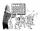Find two summands of the number 42, so that its product is minimized.
5. Last pageTwo consecutive sheets dropped out of the book. The sum of the numbers on the sides of the dropped sheets is 154. What is the number of the last page of the dropped sheets?
6. Unknown numbersThe sum of two consecutive natural numbers and their triple is 92. Find these numbers.
7. Mushrooms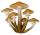For five days, we have collected 410 mushrooms. Interestingly every day we have collected 10 mushrooms more than the preceding day. How many mushrooms we have collected during 4th day?
8. Unknown numberIdentify unknown number which 1/5 is 40 greater than one tenth of that number.
9. FactoryIn the factory workers work in three shifts. In the first inning operates half of all employees in the second inning and a third in the third inning 200 employees. How many employees work at the factory?
10. Shop stores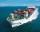Workers untied fruit to stores. In the first quarter shipments, in the second fifth shipment, in the third two-fifths of the rest and in the fourth 231 kilograms. How many fruits were together?
11. Trip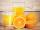On the trip drank 3/10 of pupils tea, 2/5 cola, 1/4 mineral water and remaining 3 juice. How many students were on the trip?
12. Two ages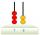Gabrielle is 9 years younger than Mikhail. The sum of their ages is 87. What is Mikhail's age?
13. Apples 2How many apples were originally on the tree, if the first day fell one third, the second day quarter of the rest and on tree remained 45 apples?
14. Tippler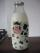Bottle with cork cost 8.8 Eur. The bottle is 0.8 euros more expensive than cork. How much is a bottle and the cork?
15. Every day 7 pagesAdelka reads the book every day 7 pages. When she reads one more page a day she will read it three days earlier. How long will Adelka read a book? How much does a book of pages have?
16. Game 27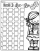Susan wanted to play the game. In the beginning, the first says a number from 1 to 8. Then the second player adds a number from 1 to 5 and tells the sum. Again, the Susan adds a number from 1-5 and say sum and etc. . . The winner must say the number 27. Wh
17. Combine / add termCombine like terms 4c+c-7c